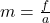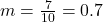Question

Coach Farris demonstrates how to serve a volleyball. She hits the volleyball with a force of 7 N and the volleyball accelerates at 10.0 m/s2. What is the mass of the volleyball? Record
your answer below. Be sure to use correct place value (2 decimal places).

1.thnahdat

## 0.70 kg

Explanation:

The mass of the volleyball can be found by using the formulaf is the force

a is the acceleration

From the question we haveWe have the final answer as

### 0.70 kg

Hope this helps you